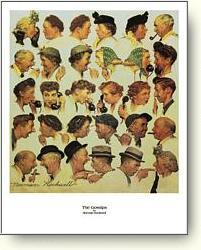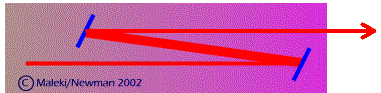### Questions on Waves

1. What is a wave?Rumors (a la Norman Rockwell)

1. What is the difference between a rumor and a physical wave?
2. Is a "heat wave" a physical wave?
3. What is a transverse wave? What is a longitudinal wave? Give an example of each.
4. How does a sound you make travel in air? How does it travel in a "cup telephone"?
5. What is wavelength? How is it related to the speed and frequency?
6. What is interference effect? Give an example of it.
7. Could waves of different amplitude interfere? How about waves of different frequency?
8. What is a standing wave?
9. What are beats? How are they generated?
10. What is the diffraction effect? When is it most pronounced?
11. What happens in diffraction from an aperture when its size decreases?  increases?